+

# Properties of Exponents

Author: Sophia Tutorial
##### Description:

Simplify an expression using the properties of exponents.

(more)

Sophia’s self-paced online courses are a great way to save time and money as you earn credits eligible for transfer to many different colleges and universities.*

No credit card required

37 Sophia partners guarantee credit transfer.

299 Institutions have accepted or given pre-approval for credit transfer.

* The American Council on Education's College Credit Recommendation Service (ACE Credit®) has evaluated and recommended college credit for 32 of Sophia’s online courses. Many different colleges and universities consider ACE CREDIT recommendations in determining the applicability to their course and degree programs.

Tutorial
what's covered
1. Properties of Exponents
1. Product Property
2. Product Property
3. Quotient Property
4. Power of Power Property
5. Power of a Quotient Property

# 1. Properties of Exponents

Problems with exponents can often be simplified using a few basic exponent properties. Exponents represent repeated multiplication. We will use this fact to discover important properties.

did you know
The word exponent comes from the Latin "expo" meaning "out of" and "ponere" meaning "place." While there is some debate, it seems that the Babylonians living in present day Iraq were the first to do work with exponents (dating back to the 23rd century BC or earlier!)

1a. Product Property of Exponents

A quicker method to arrive at our answer would have been to just add the exponents. This is known as the product property of exponents.

formula
Product Property of Exponents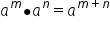The important thing here is that the expressions must have the same base. If exponential expressions with the same base are multiplied together, we can add the exponents. Here is another example: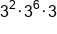Same base, add the exponents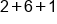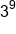Our Solution

1b. Quotient Property of Exponents

Rather than multiplying, we will now try to divide with exponents.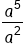Expand Exponents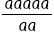Divide out two of the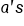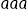Convert to Exponents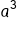Our Solution

A quicker method to arrive at the solution would have been to just subtract the exponents. This is known as the quotient property of exponents:

formula
Quotient Property of Exponents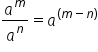Just like with the product property, it is important to note that is only holds true when the bases are the same. Here is an example: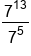Same base, subtract the exponents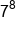Our Solution

1c. Power of a Power Property of Exponents

A third property we will look at will have an exponent raised to another exponent. This is investigated in the following example: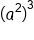This means we have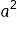three times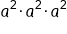Add exponents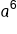Our Solution

A quicker method to arrive at the solution would have been to just multiply the exponents. This is known as the power of a power property of exponents.

formula
Power of a Power Property of Exponents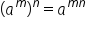This property is often combined with two other properties: power of a product, and power of a quotient. We will look at these properties next.

1d. Power of a Product Rule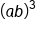This means we have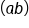three times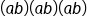Threeand three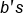can be written with exponents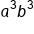Our Solution

A quicker method to arrive at the solution would have been to take the exponent of three and put it on each factor in parentheses. This is known as the power of a product property of exponents.

formula
Power of a Product Property of Exponents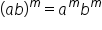hint
It is important to be careful to only use the power of a product property with multiplication inside parentheses. This property does NOT work if there is addition or subtraction. (a+b)m ≠ am + bm These are NOT equal. Beware of this error!

1e. Power of a Quotient Property of Exponents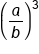This means we have the fraction three times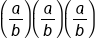Multiply fractions across the top and bottom, using exponents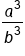Our Solution

A quicker method to arrive at the solution would have been to put the exponent on every factor in both the numerator and denominator. This is known as the power of a quotient property of exponents.

formula
Power of a Quotient Property of Exponents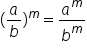The power of a power, product, and quotient properties of exponents are often used together to simplify expressions. This is shown in the following examples: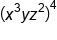Put the exponent of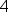on each factor, multiplying powers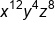Our Solution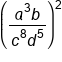Put the exponent of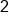on each factor, multiplying powers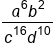Our Solution

 Product Rule of Exponents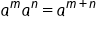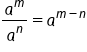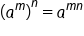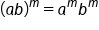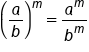summary
These five properties of exponents are often mixed up in the same problem. Often there is a bit of flexibility as to which property is used first. However, the order of operations still applies to a problem. For this reason, we suggest simplifying inside any parentheses first, then simplify any exponents (using power properties). Finally, simplify any multiplication or division (using product and quotient properties).

Source: Adapted from "Beginning and Intermediate Algebra" by Tyler Wallace, an open source textbook available at: http://wallace.ccfaculty.org/book/book.html

Formulas to Know
Power of a Power Property of ExponentsPower of a Product Property of ExponentsPower of a Quotient Property of ExponentsProduct Property of ExponentsQuotient Property of ExponentsRating